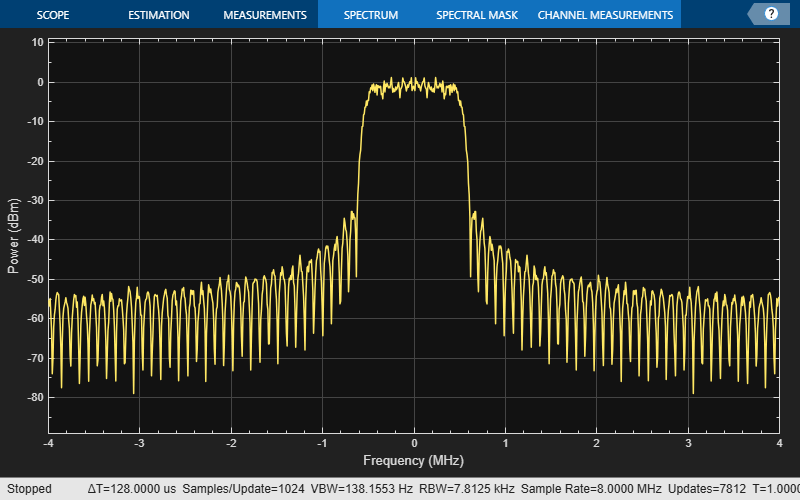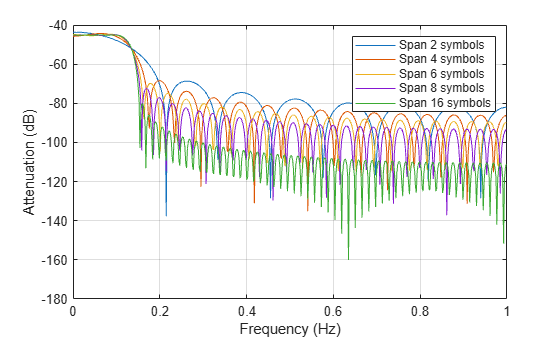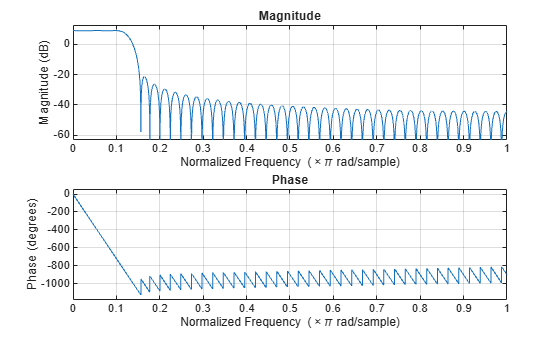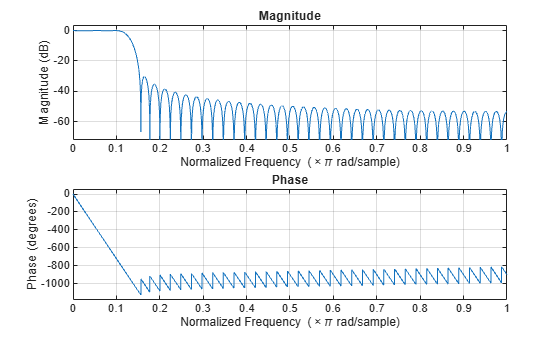comm.RaisedCosineTransmitFilter

Apply pulse shaping by interpolating signal using raised-cosine FIR filter

Description

The comm.RaisedCosineTransmitFilter System object™ applies pulse shaping by interpolating an input signal using a raised cosine finite impulse response (FIR) filter. The FIR filter has (FilterSpanInSymbols × OutputSamplesPerSymbol + 1) tap coefficients.

To apply pulse shaping by interpolating an input signal using a raised cosine FIR filter:

1. Create the comm.RaisedCosineTransmitFilter object and set its properties.

2. Call the object with arguments, as if it were a function.

Creation

Description

txfilter = comm.RaisedCosineTransmitFilter returns a raised cosine transmit FIR filter System object, which interpolates an input signal using a raised cosine FIR filter. The filter uses an efficient polyphase FIR interpolation structure and has unit energy.

example

txfilter = comm.RaisedCosineTransmitFilter(Name,Value) sets properties using one or more name-value pairs. Enclose each property name in quotes. For example, comm.RaisedCosineTransmitFilter('FilterSpanInSymbols',15) configures a raised cosine transmit filter System object with the filter span set to 15 symbols.

Properties

expand all

Unless otherwise indicated, properties are nontunable, which means you cannot change their values after calling the object. Objects lock when you call them, and the release function unlocks them.

If a property is tunable, you can change its value at any time.

Filter shape, specified as 'Square root' or 'Normal'.

Data Types: char | string

Roll-off factor, specified as a scalar in the range [0, 1].

Data Types: double

Filter span in symbols, specified as a positive integer. The object truncates the infinite impulse response (IIR) of an ideal raised-cosine filter to an impulse response that spans the number of symbols specified by this property.

Data Types: double

Output samples per symbol, specified as a positive integer.

Data Types: double

Linear filter gain, specified as a positive scalar. The object designs a raised-cosine filter that has unit energy and then applies the linear filter gain to obtain final tap coefficient values.

Data Types: double

Usage

Description

example

y = txfilter(x) applies pulse shaping by interpolating an input signal using a raised cosine FIR filter. The output consists of interpolated signal values.

Input Arguments

expand all

Input signal, specified as a column vector or a Ki-by-N matrix. Ki is the number of input samples per signal channel, and N is the number of signal channels.

For a Ki-by-N matrix input, the object processes columns of the input matrix as N independent channels.

Data Types: double | single
Complex Number Support: Yes

Output Arguments

expand all

Output signal, returned as a column vector or a Ko-by-N matrix. Ko is equal to Ki × OutputSamplesPerSymbol. Ki is the number of input samples per signal channel, and N is the number of signal channels.

The object interpolates and filters each channel over the first dimension and then generates a Ko-by-N output matrix. The output signal is the same data type as the input signal.

Object Functions

To use an object function, specify the System object as the first input argument. For example, to release system resources of a System object named obj, use this syntax:

release(obj)

expand all

 info Information about filter System object coeffs Coefficients for filters cost Computational cost of implementing filter System object freqz Frequency response of discrete-time filter fvtool Plot frequency response of filter grpdelay Group delay response of discrete-time filter impz Impulse response of discrete-time filter order Order of discrete-time filter System object
 step Run System object algorithm release Release resources and allow changes to System object property values and input characteristics reset Reset internal states of System object

Examples

collapse all

Interpolate a signal using square-root-raised-cosine (SRRC) transmit filter object and display the spectrum of the filtered signal.

Create random bipolar symbols at a symbol rate of 1e6 symbols per second.

data = 2*randi([0 1],1e6,1) - 1;

Create a SRRC transmit filter object. The default sets the filter to a square-root shape and the number of samples per symbol to 8.

txfilter = comm.RaisedCosineTransmitFilter
txfilter =
comm.RaisedCosineTransmitFilter with properties:

Shape: 'Square root'
RolloffFactor: 0.2000
FilterSpanInSymbols: 10
OutputSamplesPerSymbol: 8
Gain: 1

Filter the data by using the SRRC filter.

filteredData = txfilter(data);

Create a spectrum analyzer object with an 8e6 sampling rate. This sampling rate matches the sampling rate of the filtered signal.

spectrumAnalyzer = dsp.SpectrumAnalyzer('SampleRate',8e6);

View the spectrum of the filtered signal by using the spectrum analyzer object.

spectrumAnalyzer(filteredData)Create interpolated signals from a square-root-raised-cosine (SRRC) filter with various filter spans. Examine the magnitude response of the various filter designs.

Create SRRC filter objects setting various filter spans. Use the coeffs object function to obtain the filter coefficients.

txfilt2 = comm.RaisedCosineTransmitFilter('FilterSpanInSymbols',2);
txfilt4 = comm.RaisedCosineTransmitFilter('FilterSpanInSymbols',4);
txfilt6 = comm.RaisedCosineTransmitFilter('FilterSpanInSymbols',6);
txfilt8 = comm.RaisedCosineTransmitFilter('FilterSpanInSymbols',8);
txfilt16 = comm.RaisedCosineTransmitFilter('FilterSpanInSymbols',16);

taps2 = coeffs(txfilt2).Numerator;
taps4 = coeffs(txfilt4).Numerator;
taps6 = coeffs(txfilt6).Numerator;
taps8 = coeffs(txfilt8).Numerator;
taps16 = coeffs(txfilt16).Numerator;

Launch the filter visualization tool to show the impulse response. Specify a sample rate of 1 kHz. Display the two-sided centered response.

h = fvtool(taps2,1,taps4,1,taps8,1,taps16,1);
h.Fs = 1e3;
h.FrequencyRange = '[-Fs/2, Fs/2)';
legend('Span 2 symbols','Span 4 symbols', ...
'Span 8 symbols','Span 10 symbols')
title('Magnitude Response (dB) for Various Filter Spans')Create a square-root-raised-cosine (SRRC) transmit filter System object, and then plot the filter response. The results show that the linear filter gain is greater than unity. Specifically, the passband gain is greater than 0 dB.

txfilter = comm.RaisedCosineTransmitFilter;
fvtool(txfilter)Obtain the filter coefficients by using the coeffs object function and adjust the filter gain to unit energy.

b = coeffs(txfilter);

Because a filter with unity passband gain must have filter coefficients that sum to 1, set the linear filter gain to the inverse of the sum of the filter tap coefficients, b.Numerator.

txfilter.Gain = 1/sum(b.Numerator);

Verify that the resulting filter coefficients sum to 1.

bNorm = coeffs(txfilter);
sum(bNorm.Numerator)
ans = 1.0000

Plot the filter frequency response again. The results now show that the passband gain is 0 dB, which is unity gain.

fvtool(txfilter)